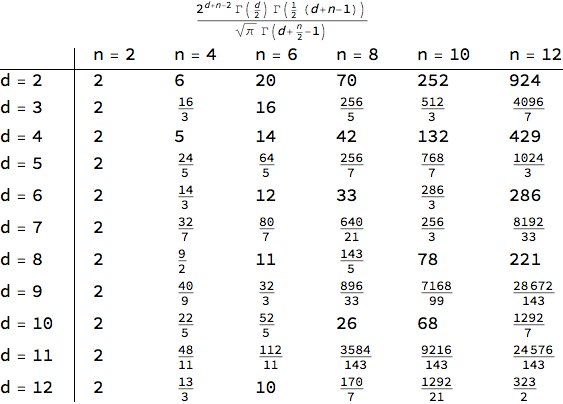I asked if we got other interesting sequences of numbers by looking at the moments for the distance of two randomly chosen points on the sphere in other dimensions.

[Greg Egan, with his usual unstoppable force](https://twitter.com/gregeganSF/status/1015393738003132417), worked out a chart of the \\(n\\)th moment of the distance of two randomly chosen points on the unit sphere in \\(\mathbb{R}^d\\), at least when \\(n\\) is even:He wrote:

> These are the nth moments for even n, for dimensions 2 to 12. So generally these are not all integers.

You can see the Catalan numbers on the \\(d = 4\\) row. But what else do you see?